# Ex 12.4 Q1 Algebraic Expression Solution- NCERT Maths Class 7

Go back to  'Ex.12.4'

## Question

Observe the patterns of digits made from line segments of equal length. You will find such segmented digits on the display of electronic watches or calculators.

(a)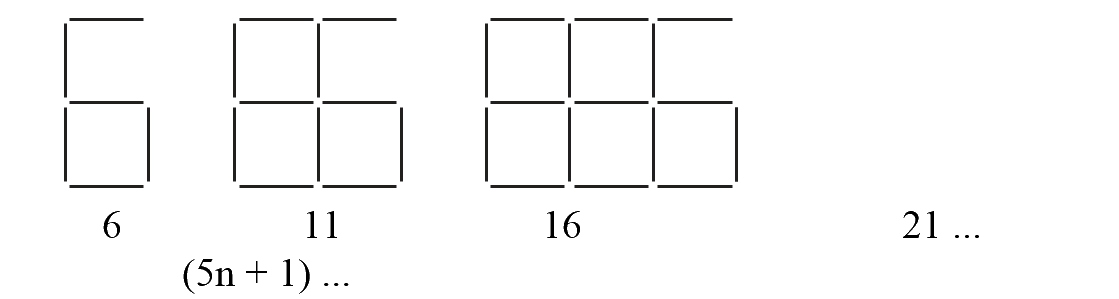(b)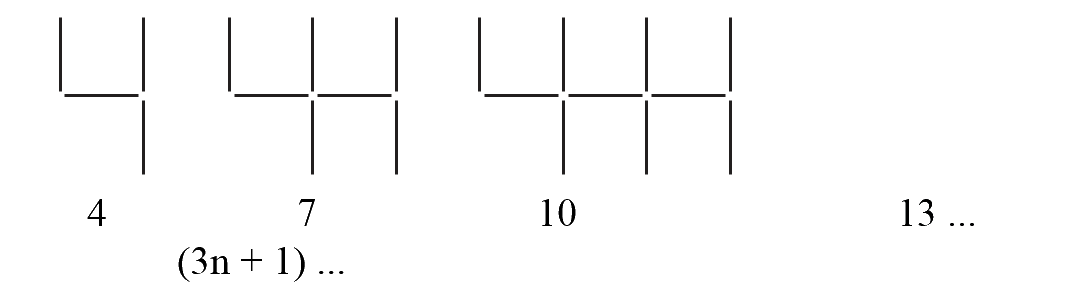(c)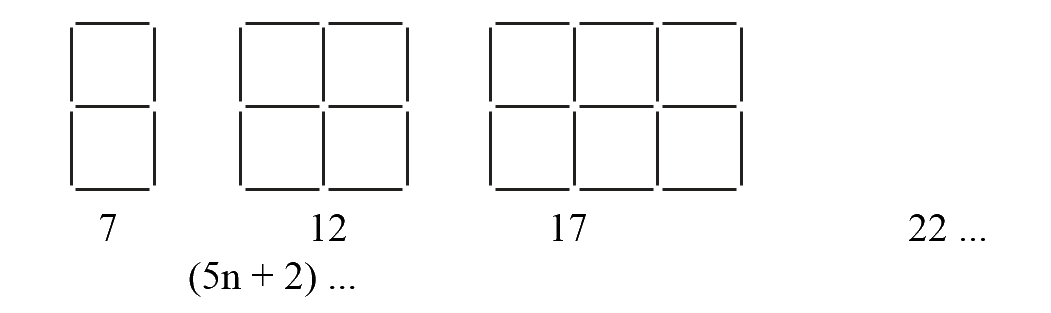If the number of digits formed is taken to be n, the number of segments required to form n digits is given by the algebraic expression appearing on the right of each pattern. How many segments are required to form $$5, 10, 100$$ digits of the kind,Video Solution
Algebraic Expressions
Ex 12.4 | Question 1

## Text Solution

What is known?

The patterns of digits made from line segments of equal length

What is unknown?

Number of segments required to form $$5, 10, 100$$ digits of the kind, ,Reasoning:

This question is very easy like simply put the value of n in the pattern formulae and you can easily find out the number of segments.

Steps:

Putting value of $$n=5, 10$$ and $$100$$ in the pattern formulae.

$$({\rm{i}})\quad 5n + 1$$ \begin{align}5 \times 5 + 1 & = 25 + 1\\& = 26\\5 \times 10 + 1 & = 50 + 1\\& = 51\\5 \times 100 + 1 & = 500 + 1\\& = 501\end{align}

$${\rm{(ii)}}\quad 3n + 1$$ \begin{align}3 \times 5 + 1 & = 15 + 1\\& = 16\\3 \times 10 + 1 &= 30 + 1\\& = 31\\3 \times 100 + 1 & = 300 + 1\\& = 301\\\end{align}

$$( {{\rm{iii}}}) \quad 5n + 2$$  \begin{align}\\5 \times 5 + 2 & = 25 + 2\\&= 27\\5 \times 10 + 2 & = 50 + 2\\& = 52\\5 \times 100 + 2 & = 500 + 2\\& = 502\\\end{align}

 S. No. Symbol Digit’s Number Pattern’s Formulae No. of. Segments (i)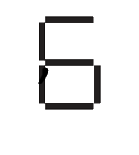$$5$$ $${\rm{5 n + 1}}$$ 26 $$10$$ 51 $$100$$ 501 (ii)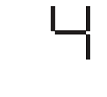$$5$$ $${\rm{3}}\,{\rm{n + 1}}$$ 16 $$10$$ 31 $$100$$ 301 (iii)$$5$$ $${\rm{5 n + 2}}$$ 27 $$10$$ 52 $$100$$ 502

Learn from the best math teachers and top your exams

• Live one on one classroom and doubt clearing
• Practice worksheets in and after class for conceptual clarity
• Personalized curriculum to keep up with school